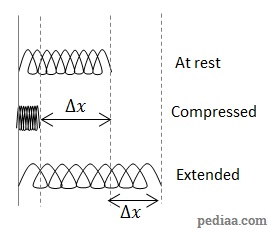# Difference Between Gravitational Potential Energy and Elastic Potential Energy

## Main Difference – Gravitational Potential Energy vs. Elastic Potential Energy

Gravitational potential energy and elastic potential energy are two different forms of energies that a particle can have. The main difference between gravitational potential energy and elastic potential energy is that the origin of gravitational potential energy is the gravitational forces acting between two massive bodies whereas the origin of elastic potential energy is the electrostatic forces between molecules that make up a material.

## What is Gravitational Potential Energy

Gravitational potential energy is the energy that an object has due to its position in a gravitational field. The gravitational potential energy is always relative: it is a comparison between the amounts of energy that an object has at different points in a gravitational field.

Imagine lifting an object with mass$m$ through a height of$h$ in a uniform gravitational field. The weight of the object is given by$mg$. If the object is lifted at a constant speed so that all the work done from lifting the object is given to the object as gravitational potential energy, we can calculate the gain in gravitational potential energy of the body due to its rise. Since the object is lifted at a constant speed, the forces are balanced and lifting force would be$F=mg$. Since the object is raised through a height$h$, the work done is given by$mgh$. This means that the gain in gravitational potential energy is given by:$\Delta E_{gravitational}=mgh$

## What is Elastic Potential Energy

An object gains elastic potential energy if it is physically deformed. When an object is deformed, the molecules that form the material are forced to move away from their equilibrium positions. If the material is elastic, then the molecules try to get back to their equilibrium positions. This gives the material an ability to do work. Therefore, when an elastic material is deformed, we say that the material has elastic potential energy. For instance, elastic potential energy is gained by a spring/rubber band when it is stretched.Rubber bands, when stretched, gain potential energySprings: at rest, compressed and extended

Think about compressing/extending the spring. The more deformed a spring is, the more is the force needed to deform it further. The force increases with extension:

The work done on the spring is given by the area under the force vs. extension graph. In this case, the area is given by$\frac{1}{2}F\Delta x$. According to Hooke’s law,$F=k\Delta x$ so the total work done to compress or extend the spring by$\Delta x$ is$\frac{1}{2}k{\Delta x}^2$, where$k$ is the spring constant of the spring. If there are no other forces, then all the work done on the spring would be converted to elastic potential energy$E_{el}$ in the spring. Then,$E_{el}=\frac{1}{2}k{\Delta x}^2$

## Difference Between Gravitational Potential Energy and Elastic Potential Energy

### Definition:

Gravitational potential energy is the energy that an object has due to its position in a gravitational field.

Elastic Potential Energy is the energy that a material gains after undergoing deformation.

### How it is formed:

Gravitational potential energy originates from the gravitational attraction between masses.

Elastic potential energy is due to electrostatic repulsion between atoms and molecules making a material.

### What is does:

Gravitational potential energy attempts to pull the centres of masses of objects as close as possible.

Elastic potential energy attempts to keep atoms and molecules making up an object at a certain separation where these particles can maintain an equilibrium.

Image Courtesy:

Untitled image by Booyabazooka at PublicDomainPictures [Public Domain], via Pixabay